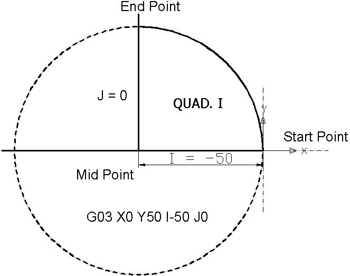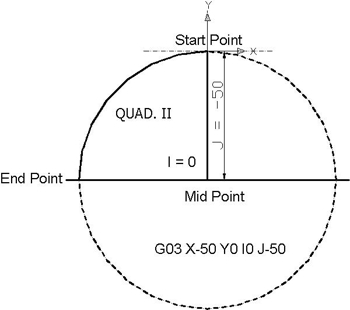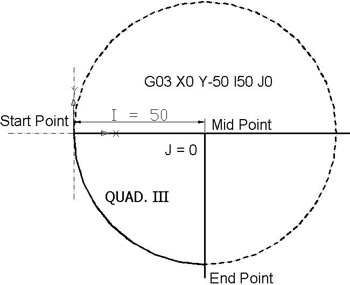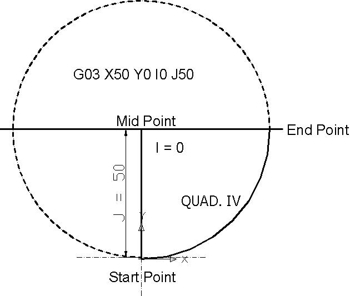# Circular Interpolation Concepts & Programming Part 3 (Use of I J K)

3rd part of Multi-Series Articles “Circular Interpolation Concepts & Programming”.
This part briefly explains how cnc machinists can Calculate and Program circular interpolation G02 G03 with the use of I J and K.

## Circular Interpolation G02 G03 I, J, K Concepts & Programming

The axis of the Arc must be parallel to the X-, Y- or Z-axis of the machine coordinate system. The axis or the plane perpendicular to the axis is selected with G17 (Z-axis, XY-plane), G18 (Y-axis, XZ-plane) or G19 (X-axis, YZ-plane). I, J and K are the offsets from the current location. At one time only two of I, J, and K will be used. This will depend on what arc plane has been selected

• G17 – Use I and J
• G18 – Use I and K
• G19 – Use J and K

The I, J and K arguments specify the DISTANCE from the ARC START POINT to the CENTER POINT of the arc. Note that the start point of the arc is NOT GIVEN in a G02 or G03 command. The start point is determined by the location of the cutter when the command is implemented. Also, the center point is never given explicitly in the command. I, J, and K are DISTANCES. If the geometry of the circle is impossible (to within .0001), an error is usually thrown.

The following figure shows the four quadrants of circle and I, J calculation from start point to end point. Circle radius is 50 mm.Quad I from 0⁰ to 90⁰ – Circular Interpolation Concepts

 Quad I from 0⁰ to 90⁰ Start Point X50 Y0 End Point X0 Y50 I -50 J 0 G03 X0 Y50 I-50 J0Quad II from 90⁰ to 180⁰ – Circular Interpolation Concepts

 Quad II from 90⁰ to 180⁰ Start Point X0 Y50 End Point X-50 Y0 I 0 J -50 G03 X-50 Y0 I0 J-50Quad III from 180⁰ to 270⁰ – Circular Interpolation Concepts

 Quad III from 180⁰ to 270⁰ Start Point X-50 Y0 End Point X0 Y-50 I 50 J 0 G03 X0 Y-50 I50 J0Quad IV from 270⁰ to 0⁰ – Circular Interpolation Concepts

 Quad IV from 270⁰ to 0⁰ Start Point X0 Y-50 End Point X50 Y0 I 0 J 50 G03 X50 Y0 I0 J50